# Bring your own hyperparameter optimization algorithm on Amazon SageMaker

In this blog post, we’ll discuss how to implement custom, state-of-the-art hyperparameter optimization (HPO) algorithms to tune models on Amazon SageMaker. Amazon SageMaker includes a built-in HPO algorithm, but provides the flexibility to use your own HPO algorithm. We’ll provide you with a framework to incorporate an HPO algorithm that you choose. However, before we do this, let’s review some basics.

Four common steps in any machine learning (ML) pipeline, regardless of the framework, are build, train, tune and deploy. In the build stage, data is being collected, massaged and prepared for ML training, and an algorithm is being written from scratch, or using a popular ML framework. Next, a model is trained by pointing the algorithm to the prepared data, and incrementally improving some performance metric (like validation accuracy). After the model is trained to a desired level of accuracy, it is ready to be hosted or deployed for use in a larger ML architecture. Before training begins, each algorithm is initiated with a set of values, called hyperparameters, that define various aspects of the algorithm. For instance, the popular Xgboost algorithm (or Extreme Gradient Boosted Trees), also provided as a built-in algorithm in Amazon SageMaker, has the following parameters that need to be set prior to training the model, and along with the data, completely decides how the training run will progress and what the final accuracy of the model will be.

 Parameter Name Parameter Type Recommended Ranges alpha Continuous MinValue: 0, MaxValue: 1000 colsample_bylevel Continuous MinValue: 0.1, MaxValue: 1 colsample_bytree Continuous MinValue: 0.5, MaxValue: 1 eta Continuous MinValue: 0.1, MaxValue: 0.5 gamma Continuous MinValue: 0, MaxValue: 5 lambda Continuous MinValue: 0, MaxValue: 1000 max_delta_step Integer [0, 10] max_depth Integer [0, 10] min_child_weight Continuous MinValue: 0, MaxValue: 120 num_round Integer [1, 4000] subsample Continuous MinValue: 0.5, MaxValue: 1

Each parameter in this table can either take float (parameter type “Continuous”), or integer values (parameter type “Integer”), and can be set prior to training. Typically, the user chooses the values of these parameters by experience, or worse, by randomly guessing values in the recommended ranges. If only there was an automated process that can tell me what the best choices for values of these variables, and also provide me with a trained model that has the best performance … Enter hyperparameter optimization (HPO)!

HPO is a family of methods that tries different choices of hyperparameters to determine which is the optimum choice of parameters that defines the training job. This optimum choice of parameters corresponds to the best model according to some metric like validation accuracy. For a good review of HPO applied to Machine Learning, please see Claesen and De Moor, 2015 (https://arxiv.org/abs/1502.02127) The two most intuitive methods used in HPO are grid search and random search. In “grid search,” we run training jobs for each combination of parameter values by running through every value of every variable given some lower and upper bound (or range) of the variable to be explored. For float values, we also choose a resolution (for example 0.5), and this decides how many points in the dimension of that float parameter we wish to evaluate. A HPO problem with 11 parameters to tune as shown earlier is an 11 dimensional optimization problem with the objective of maximizing validation accuracy. As you can imagine, grid search is extremely expensive, especially if there are several combinations of float and integer parameter choices to explore. On the other hand, “random search” picks random values of these parameters within acceptable ranges of parameter values, and runs training jobs. Given a maximum number of training jobs as a budget, you can explore multiple random combinations and pick the training job that has the best performance. Both random search and grid search explore the design space of this problem well, but are not efficient. Also, they do not continuously improve the value of the objective function (in this example, validation accuracy). Fortunately, decades of optimization research is here to help!

Any optimization problem can be thought of as finding the values of the design variables x that optimize (meaning, minimize or maximize) the value of an objective function f. Assume that this objective function is f is (x-1)^2, and our aim is to find the minimum value of this function for all real values of x. It is easy to see that the minimum value of f is 0, and the value of x at this mimimum, or x* is 1, since (1-1)^2 = 0. In many cases, the function f is a value that is returned after a process that does not have a closed form representation like (x-1)^2. These functions are called black-box functions, since we are blind to the procedure to calculate the value of f, but we have a mechanism to “send in” a value of x to this function and wait for it to “return” a value. In HPO, the black-box function f is the value of a metric (like accuracy) returned at the end of an ML training job, and x is the set of hyperparameters that defines the training job.

## Brief  review of state-of-the-art HPO algorithms

There are a large number of HPO algorithms that exist in the literature, ranging from biologically inspired algorithms and kernel methods to ensemble models. Some algorithms are suited for standard optimization problems where the objective function can be evaluated instantaneously. For example, calculating the value of (x-1)^2 given a value of x is trivial, and takes less than a second to evaluate. However, the “function” we are trying to optimize in HPO is an entire ML training job! ML training jobs can take from minutes to hours to complete. These kinds of problems fall under the category of expensive, black-box optimization problems. They are “expensive” since each objective function is costly and time consuming to evaluate since it depends on the completion of an entire training job. They are “black-box” because the training job cannot be expressed as a closed-form or algebraic equation. Machine Learning HPO problems are different from typical black-box optimization problems in the following ways:

1. ML training jobs can take varying amounts of time, even for slightly different hyperparameter values, and
2. ML training jobs are themselves optimization problems!

Bayesian optimization techniques are some of the most efficient approaches in terms of the number of function evaluations required to optimize a function, and has been shown to outperform other state of the art global optimization algorithms on a number of challenging optimization benchmark functions. Bayesian Optimization, and other black-box optimization algorithms are useful when objective function evaluations are costly, when you don’t have access to derivatives, or when the problem at hand is non-convex.

## The Amazon SageMaker built-in automatic model tuning algorithm

Amazon SageMaker automatic model tuning uses an algorithm called Bayesian Optimization  to choose the hyperparameter values that result in a model that performs the best, as measured by a metric that you choose (https://docs.aws.amazon.com/sagemaker/latest/dg/automatic-model-tuning-how-it-works.html).

A detailed discussion on Bayesian Optimization is given by Brochu et al. https://arxiv.org/abs/1012.2599). When choosing the best hyperparameters for the next training job, HPO on Amazon SageMaker considers everything it knows about this problem so far. It chooses values of hyperparameters that are most likely to produce an incremental improvement on the best result found so far. This allows hyperparameter tuning to explore the design space, and also focus on (or “exploit”) better areas of the design space. Other times it chooses a set of hyperparameters far removed from those it has tried. This allows it to explore the space to try to find new areas that are not well understood. Bayesian Optimization is a great choice for HPO since it can be applied to search spaces that involve categorical or conditional inputs, or even combinatorial search spaces with multiple categorical inputs and solved efficiently. Evaluating the objective function in this case means running a training job with a set of hyperparameters x and recording a value of the accuracy (or some other metric) from the training job. Bayesian Optimization tries successive values of x to update its understanding of the objective function f; in Bayesian Optimization, this is called a probabilistic surrogate model.

The figure below sourced from Shahriari et. al. (https://ieeexplore.ieee.org/stamp/stamp.jsp?tp=&arnumber=7352306) shows two initial observations (black dots) in the “n=2” subplot, where ‘n’ denotes the total number of actual function evaluations. The black dots represent the actual measurements of the objective metric by directly evaluating the objective (in our case, by running a training job and measuring the value of f). The blue shaded region shows a region of uncertainty between actual points of measurements (black dots) since we haven’t actually evaluated f at these points. Bayesian Optimization uses an acquisition function (more details in the paper cited earlier) to decide where the next experiment should be conducted – i.e., where the next function evaluation should take place. This new point is shown as a red dot in the image below. Notice how the shapes of the probabilistic surrogate model (in blue) changed after the addition of a new point. This process continues until the minimum of the actual objective function is reached, and the optimum is found.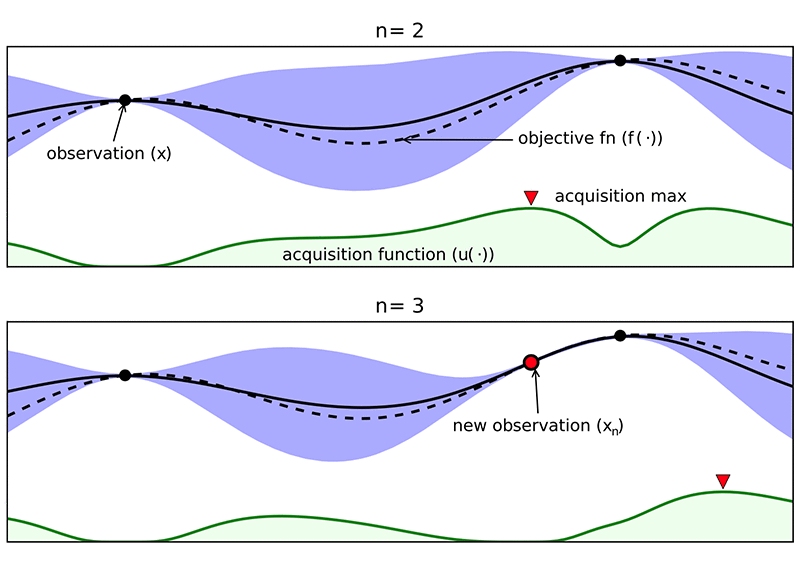The explore/exploit trade-off is commonly seen in many optimization approaches, especially a class of optimization algorithms called “evolutionary optimization” algorithms that excel in black-box optimization and gradient-free optimization.

Why use evolutionary optimization methods (or any method other than Bayesian Optimization)?

Evolutionary optimization has been used in varying large scale, black-box and gradient-free application problems. While Bayesian Optimization is one of the methods that can help users find the optimal model, there are many other methods that can be used in the optimization process. Evolutionary optimization methods are typically highly parallelizable and very intuitive, since they are inspired by processes that occur in nature, like survival of the fittest. Hundreds of other optimization algorithms are published every year in peer reviewed journals, and go through rigorous testing against standard benchmark algorithms, and are only published if they perform better than existing state of the art algorithm. Given this sheer pace of research in the optimization community, it is important to provide the flexibility to try out new algorithms in the perspective of HPO.

As we mentioned earlier, the main purpose of this blog is to provide a framework to allow you to incorporate an HPO algorithm that you choose. As examples, we’ll be using the Amazon SageMaker built-in HPO algorithm, random search, and some popular evolutionary optimization algorithm.

## Evolutionary Optimization Approaches

Evolutionary Optimization, as the name suggests, is inspired by the process of natural evolution. The theory of evolution by natural selection can be summarized as follows:

• Successive generations of individuals can differ from previous ones. Children inherit characteristics of their parents. But combining and mutating these characteristics introduces variation from generation to generation.
• Individuals in the population are often represented their DNA. In practice, this “DNA” of an individual (here, a single training job) has to be in encoded as a bit string and decoded again when needed.
• Less fit individuals (here, training jobs) are selectively eliminated (‘survival of the fittest’).

The algorithm itself makes sure that individuals in successive generations to perform better than those in previous ones overall. In the rest this blog post, we will be using the MNIST dataset as an example to explore various custom HPO strategies.

## Problem description (MNIST image classification)

The Modified National Institute of Standards and Technology or MNIST dataset contains images of handwritten digits from 0 to 9 and is a popular ML problem (https://en.wikipedia.org/wiki/MNIST_database). The MNIST dataset contains 60000 training images and 10000 test images. A few of these images are shown below.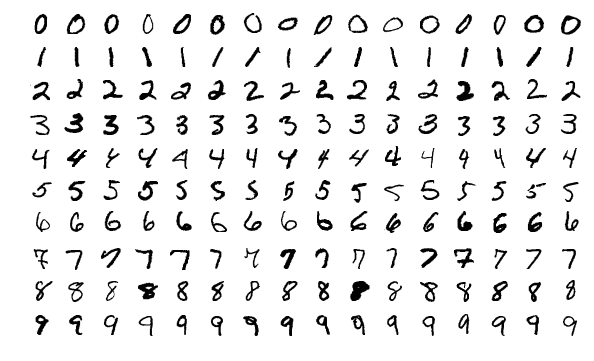In our example, we will be following an example provided out of the box in Amazon SageMaker that can be found here: https://github.com/awslabs/amazon-sagemaker-examples/tree/master/hyperparameter_tuning/mxnet_mnist

This notebook describes how you can set up the data in Amazon S3, and use the built-in HPO algorithm provided by Amazon SageMaker. Each training job uses a custom MXNet script that defines a multi-layer neural network. The training script uses the hyperparameter values that the HPO job defines for each training job.

You can download the notebook and python files for this tutorial from here.

There are several reasons why you may want to BYO HPO:

1. The “No Free Lunch Theorem” (Wolpert and Macready 1997) which states that for any search/optimization algorithm, any elevated performance over one class of problems is exactly paid for in performance over another class. In other words, there is no “best” HPO algorithm, and each algorithm has certain characteristics that help solve the problem at hand better. For instance, random search is highly parallelizable and may perform better than other algorithms for some problems in terms of performance.
2. State-of-the-Art – ML and optimization are extremely fast moving research communities; There are now algorithms that are pitched against each other in international competitions to solve never-before-solved-problems, especially problems that are extremely difficult to solve such as ones with extremely noisy function spaces and a 100 million design variables… yes you read that right (see IEEE Congress on Evolutionary Computation (CEC) for details on various such competitions! https://ieeexplore.ieee.org/document/7744241 ). By comparison, what we will be tuning in our example in this blog will have only 2 variables.
3. In-house or home-bred algorithm – you may have your own, proprietary HPO algorithm that you want to test.

### Random search

First, let’s explore random search as an HPO strategy. As a reference, we use this recently published blog post and make a few changes to suit our problem: https://aws.amazon.com/blogs/machine-learning/amazon-sagemaker-automatic-model-tuning-produces-better-models-faster/

As with all training notebooks, our example provided here starts with getting an Amazon SageMaker role. We then upload the train and test data to Amazon S3:

``````region = boto3.Session().region_name
train_data_location = 's3://sagemaker-sample-data-{}/mxnet/mnist/train'.format(region)
test_data_location = 's3://sagemaker-sample-data-{}/mxnet/mnist/test'.format(region)``````

Similar to training a single MXNet job in Amazon SageMaker, we define our MXNet estimator passing in the MXNet script, IAM role, (per job) hardware configuration, and any hyperparameters we’re not tuning.

``````estimator = MXNet(entry_point='mnist.py',
role=role,
train_instance_count=1,
train_instance_type='ml.m4.xlarge',
sagemaker_session=sagemaker.Session(),
base_job_name='DEMO-hpo-mxnet',
hyperparameters={'batch_size': 100})``````

‘mnist.py’ is the script that defines functions that load the data from S3, builds the model neural network using MXNet, and trains the model. In terms relevant to this random search section as well as the following Evolutionary Algorithm sections, the estimator here is also the “individual” in the “population” that we will be evaluating using a metric, validation accuracy. Generations of these individuals will successively improve and we’ll finally end up with an optimum training job definition. Each training job is defined by a set of hyperparameters, which fall within a predetermined hyperparameter range:

``````hyperparameter_ranges = {'learning_rate': ContinuousParameter(0.01, 0.2),
'num_epoch': IntegerParameter(10, 50)}
``````

In addition to a static hyperparameter (batch size = 100), we have two other hyperparameters that we want to tune – learning rate (“learning_rate”), which can take any float value between 0.01 and 0.2, and number of epochs (“num_epoch”), which can take any integer value between 10 and 50. In practice, the length of this list is much larger, but we’ll be using these three parameters in this example for simplicity.

We’ll construct the random search function to look similar to the built-in HPO tuner function in SM, which looks like this:

``````tuner = HyperparameterTuner(estimator,
objective_metric_name,
hyperparameter_ranges,
metric_definitions,
max_jobs=9,
max_parallel_jobs=3)``````

We reuse random_tuner.py file and included functions used in https://aws.amazon.com/blogs/machine-learning/amazon-sagemaker-automatic-model-tuning-produces-better-models-faster/

Here are brief descriptions of some important classes and functions in the file:

• Classes StaticParameter, ContinuousParamater, IntegerParameter, and CategoricalParameter initialize hyperparameters that belong to these classes with appropriate values from given hyperparameter ranges. These classes have a method called get_value() that returns random values of that particular hyperparamter, while still adhering to that hyperparameter’s acceptable range (lower and upper bound).
• The _get_random_hyperparameter_values() uses these classes to generate a hyperparameter set for each training job.
• The random_search() runs some number of (“max_parallel_jobs”) jobs in parallel per “generation”.

This generation parameter is where this implementation of the random tuner differs from the blog post cited earlier. This is so that we can continue our discussion seamlessly into the meaning of generation for other evolutionary optimization algorithms. Let’s assume you want to run 10 sets of jobs, with each set performing 3 jobs in parallel. Each set is a generation, and each training job is a population member in that generation.

After deploying max_parallel_jobs number of training jobs, we wait for these jobs to complete (using the boto3 Amazon SageMaker describe_training_job function) and then start the next generation. Note that there is no relation between successive generations. That is to say that individuals in the next generation may not improve, since hyperparameters are set completely randomly. This is the primary difference when compared to evolutionary algorithms.

After all the jobs are completed, we use the helper function table_metrics(). Internally, CloudWatch logs are parsed to find the final validation accuracy of each job, and then presented in the following sorted tabular format:

``````random_metrics = rt.table_metrics(jobs, rt.get_metrics(jobs, 'Validation-accuracy=([0-9\\.]+)'))
random_metrics.sort_values(['objective'], ascending=False)``````

This can also be done using SageMaker Search. For more information, see https://docs.aws.amazon.com/sagemaker/latest/dg/API_Search.html.

Of several runs that used random search, the best available run had outputs as follows: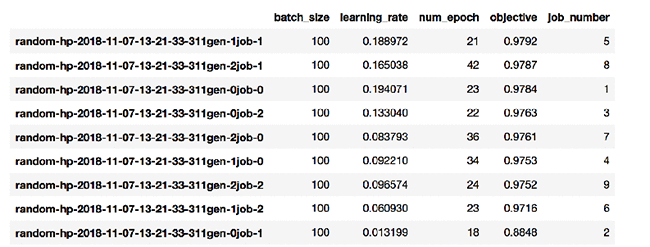As we can see, job number 5 (which corresponds to generation #1, job #1 from the job name on the leftmost row) has the highest validation accuracy of 0.9792. There is no guarantee that as more jobs are run, your overall solution will improve.

### Evolutionary search – Genetic algorithm

Genetic algorithm is an extremely common choice within the world of evolutionary search due to its ease of use, but it is certainly not the state-of-the-art (but remember the no free lunch theorem!). The following blog post provides a good introduction to Genetic Algorithm – https://towardsdatascience.com/introduction-to-genetic-algorithms-including-example-code-e396e98d8bf3. A simplified pseudocode for genetic (and other evolutionary) algorithms in general follows:

1. Generate initial population (multiple sets of hyperparameters, each hyperparameter set representing a single individual in the population)
2. Evaluate initial population (run training jobs and get objective function, e.g., validation accuracy for each job)
3. Randomly select two “parents” in the population (any two individual members), and then generate two new “children” using the combination of parents
1. In a genetic algorithm, each population member has a chromosome that is representative of the original hyperparameters. In our two variable example, the parameters we want to optimize for maximum validation accuracy are num_epochs and learning_rate. Let us “encode” these in 3-bit binary representation. num_epochs = 5 corresponds to “101” in 3-bit binary. For float values, you discretize the range of the continuous parameter, so a learning rate of 0.01 corresponds to “011” in 3 bit binary. Thus the “chromosome” that represents this individual is obtained by concatenating these two binary “genes” – “101011”. If selected as a parent, this individual combines its genes with another parent (called “crossover”) and produces a new individuals. Bits getting corrupted can also introduced diversity – this is called “mutation.”
2. Other algorithms such as differential evolution directly use the float and integer values to perform crossover and mutation.
4. Evaluate the new generation with the newly generated children (in our case, that means run all training jobs corresponding to each population member, and measure the final Validation accuracy).
5. Repeat steps 3 and 4 until the maximum number of generations is reached, or if some other stopping condition is encountered (e.g., not enough improvement in objective function).

Several popular implementations of optimization algorithms are available for practical use – for e.g., in CPLEX, GUROBI, IPOPT, SciPy, SNOPT, Pyomo etc. Here, we will be using a Python package called “inspyred” (https://pythonhosted.org/inspyred/).

Install the package using the following command in a notebook cell or the terminal:

``!pip install inspyred``

The example notebook first provides a primer on optimization using a toy function. As in the random search case, we first import the classes and functions from the provided evolutionary_tuner.py file.

``import evolutionary_tuner as et``

This file contains classes similar to the random_tuner.py file that defines the various types of hyperparameters, namely Static, Continuous, Integer, and Categorical. Typically in evolutionary optimization algorithms, we map the categorical variables to integer values. In the current example, we set the hyperparameters based on the type of hyperparameter as follows:

``````hyperparameter = {'batch_size': et.StaticParameter(100),
'learning_rate': et.ContinuousParameter(0.01, 0.2),
'num_epoch': et.IntegerParameter(10, 50)}
``````

Then, we start the tuning job using the statement:

``jobs=et.evolutionary_search(base_name=None,hyperparameters=hyperparameter,generations=3,max_parallel_jobs=6,train_fn=fit_evolutionary)``

Finally, we can call table_metrics to tabulate results of all jobs:

``````evol_metrics = et.table_metrics(jobs, et.get_metrics(jobs, 'Validation-accuracy=([0-9\\.]+)'))
evol_metrics
``````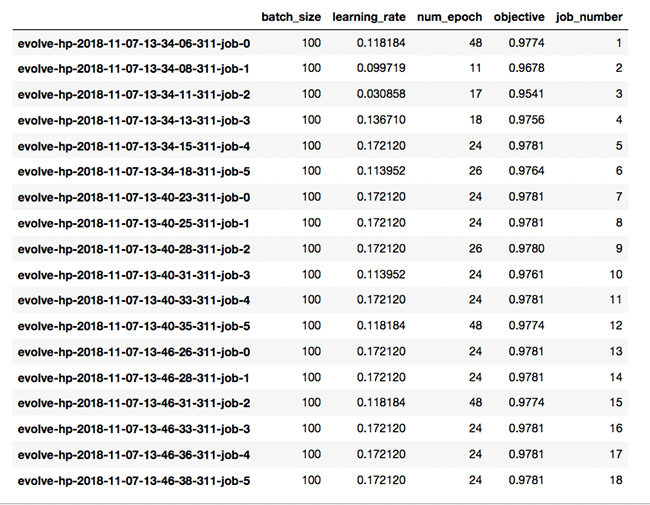Notice how we do not need to sort the results, since as each generation progresses, we are guaranteed that results will generally improve. Now, let’s dive deeper into the functions that actually perform the evolutionary optimization. First, we initialize a GA optimizer available in inspyred:

``ea = inspyred.ec.GA(prng)``

Then, we create two functions, generate_population and evaluate_population. The generate_population function generates a new generation of individuals while adhering to the hyperparameter ranges provided as an input  The evaluate_population function runs an entire generation of training jobs and waits for completion. After all jobs have been completed, it gets training job metrics from Amazon CloudWatch and returns a list of fitness values (or objective values, here validation accuracy) to the optimizer.

Based on the values of the hyperparameters for all candidates in the current generation, and the corresponding fitness values for each job, the next generation of children are spawned in the new generation for a new set of training jobs. In comparison, the build in Bayesian optimization reached a best validation-accuracy of 0.9789 after 49 epochs and a slightly different learning rate.

### Evolutionary search – Particle swarm optimization

Trying out other optimizers using this framework is very easy. All we have to change is the constructor that gets a particular algorithm from inspyred. For using particle swarm optimization, use

``ea = inspyred.swarm.PSO(prng)``

Each algorithm might have additional parameters that you may have to set. For example, here, set neighborhood_size=5.

Then call et.evolutionary_search(…) with the same arguments that are used in the genetic algorithm case. The results obtained are as follows, with a best achieved validation accuracy of 0.9795.

### Evolutionary search – Pareto archived evolution strategy (PAES)

As with PSO, first use the following to get the PAES object:

``ea = inspyred.swarm.PSO(prng)``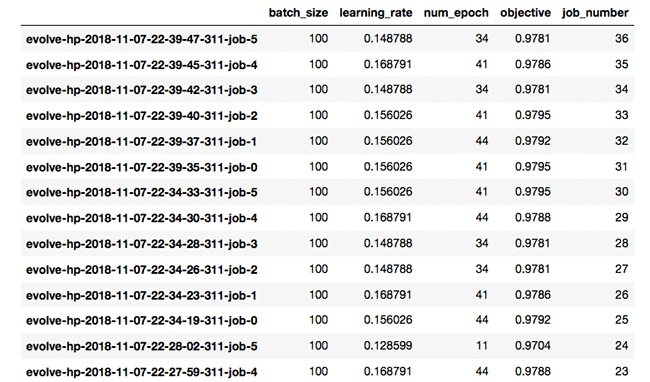With PSO, we get a slightly higher validation accuracy of 0.9795!

The change required in the constructor for running PAES is

``ea = inspyred.ec.emo.PAES(prng)``

Also set the following algorithm specific parameters:

``max_archive_size=100, num_grid_divisions=4``

Results from HPO using PAES are summarized in the following excerpt: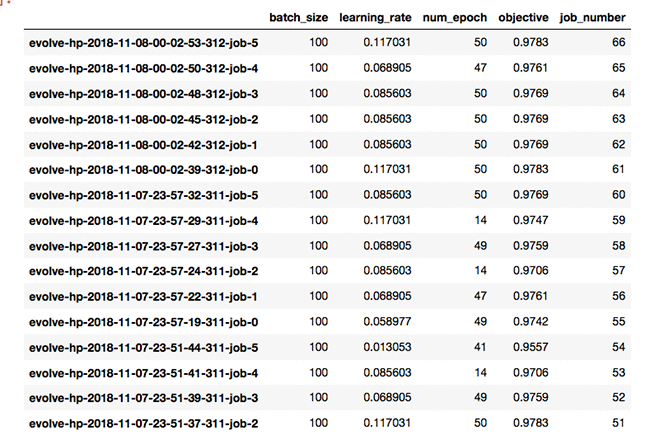Final validation accuracy was 0.9783.

In this blog, we reviewed the basics of HPO and SageMaker’s implementation, and used the flexibility of SageMaker to implement our own HPO algorithms to tune models. While we used a few algorithms from the inspyred package in this blog, some other algorithms that you can use within inspyred include evolution strategy, simulated annealing, differential evolution, estimation of distribution, non-dominated sorting genetic algorithm, and ant colony optimization. Also, for non-evolutionary algorithms based on Python libraries, check out pyomo and the optimization tools in scipy.

Finally, if you need a topic to lose some sleep over, think of the following. Given that we have explored multiple optimizers that optimize for the best training job (which are themselves optimization runs), do we now need another optimizer that chooses the best parameters for the HPO algorithms themselves?Shreyas Subramanian is a AI/ML specialist Solutions Architect, and helps customers by using Machine Learning to solve their business challenges using the AWS platform.